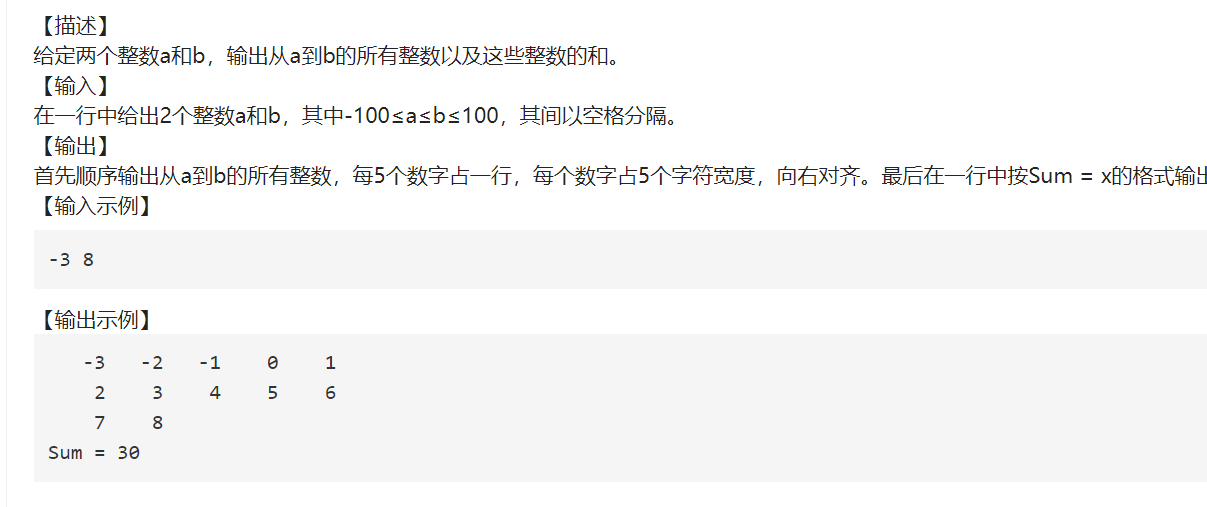浏览 34

# 关于#python#的问题：print('请输入a,b两个整数print('请输入a,b两个整数，其中-100<=a<b<100')
a,b=map(int,input().split())
sum=0
count=0
for i in range(a,b):
i+=1
print("{0: >{1}}".format(i,5))
sum+=i
count+=1
if count%5==0:
print('\n')
print('\n')
print('Sum=%d'%sum)

• 写回答

#### 悬赏问题

• ¥15 MATLAB 调用comsol如何加速设计？
• ¥15 echarts地图添加点击事件
• ¥15 openpyxl库无法全部写入文本到单元格
• ¥15 不知道带什么标题好随便搞一个吧
• ¥15 ffmpeg 图片合成视频
• ¥15 软科大学爬取获取所有数据
• ¥15 如何修改损失函数以及代码讲解
• ¥15 有偿咨询！！程序的小数点怎么取到后四位啊！
• ¥15 IRS智能反射面相关文章代码
• ¥15 landsat5的两幅影像镶嵌前波段是123457，为什么镶嵌后波段变成了123456？